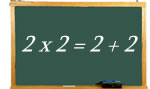Logic puzzles, riddles, math puzzles and brainteasers - pzzls.com

Vandaag is het 3 December 2023

# 2 x 2 = 2 + 2 - math puzzle

Difficulty:Rating: 2.9/5.0

 Two times two is equal to two and two. But are there more number pairs for which the sum of the numbers is is equal to the product of the numbers? The numbers may be different.